# Mapped SI Engine

Spark-ignition engine model using lookup tables

•Libraries:
Powertrain Blockset / Propulsion / Combustion Engines
Vehicle Dynamics Blockset / Powertrain / Propulsion

## Description

The Mapped SI Engine block implements a mapped spark-ignition (SI) engine model using power, air mass flow, fuel flow, exhaust temperature, efficiency, and emission performance lookup tables. You can use the block for:

• Hardware-in-the-loop (HIL) engine control design

• Vehicle-level fuel economy and performance simulations

The block enables you to specify lookup tables for these engine characteristics. The lookup tables, developed with the Model-Based Calibration Toolbox™, are functions of commanded torque, Tcmd, brake torque, Tbrake, and engine speed, N. If you select , the tables are also a function of engine temperature, TempEng.

Table Parameter Setting
`off``on`

Power

ƒ(Tcmd,N)ƒ(Tcmd,N,TempEng)

Air

ƒ(Tbrake,N)ƒ(Tbrake,N,TempEng)

Fuel

Temperature

Efficiency

HC

CO

NOx

CO2

PM

To bound the Mapped SI Engine block output, the block does not extrapolate the lookup table data.

### Virtual Calibration

If you have Model-Based Calibration Toolbox, click Calibrate Maps to virtually calibrate the 2D lookup tables using measured data. The dialog box steps through these tasks.

Description

Import firing data

Import this loss data from a file. For example, open `<matlabroot>/toolbox/mbc/mbctraining/SiEngineData.xlsx`.

Required Data

Optional Data

• Engine speed, rpm

• Engine torque, N·m

• Air mass flow rate, kg/s

• Brake specific fuel consumption, g/(kW·h)

• CO2 mass flow rate, kg/s

• CO mass flow rate, kg/s

• Exhaust temperature, K

• Fuel mass flow rate, kg/s

• HC mass flow rate, kg/s

• NOx mass flow rate, kg/s

• Particulate matter mass flow rate, kg/s

Collect firing data at steady-state operating conditions when injectors deliver the fuel. Data should cover the engine speed and torque operating range. Model-Based Calibration Toolbox uses the firing data boundary as the maximum torque.

To filter or edit the data, select . The Model-Based Calibration Toolbox Data Editor opens.

Import non-firing data

Import this non-firing data from a file. For example, open `<matlabroot>/toolbox/mbc/mbctraining/SiEngineData.xlsx`.

• Engine speed, rpm

• Engine torque, N·m

Collect non-firing (motoring) data at steady-state operating conditions when fuel is cut off. All non-firing torque points must be less than zero. Non-firing data is a function of engine speed only.

Generate response models

For both firing and non-firing data, the Model-Based Calibration Toolbox uses test plans to fit data to Gaussian process models (GPMs).

To assess or adjust the response model fit, select . The Model-Based Calibration Toolbox Model Browser opens. For more information, see Model Assessment (Model-Based Calibration Toolbox).

Generate calibration

Model-Based Calibration Toolbox calibrates the firing and non-firing response models and generates calibrated tables.

To assess or adjust the calibration, select . The Model-Based Calibration Toolbox CAGE Browser opens. For more information, see Calibration Lookup Tables (Model-Based Calibration Toolbox).

Update block parameters

Update the block lookup table and breakpoint parameters with the calibration.

### Cylinder Air Mass

The block calculates the normalized cylinder air mass using these equations.

`$\begin{array}{l}{M}_{Nom}=\frac{{P}_{std}{V}_{d}}{{N}_{cyl}{R}_{air}{T}_{std}}\\ L=\frac{\left(\frac{60s}{min}\right)Cps\cdot {\stackrel{˙}{m}}_{air}}{\left(\frac{1000g}{Kg}\right){N}_{cyl}\cdot N\cdot {M}_{Nom}}\end{array}$`

The equations use these variables.

 L Normalized cylinder air mass ${M}_{Nom}$ Nominal engine cylinder air mass at standard temperature and pressure, piston at bottom dead center (BDC) maximum volume, in kg $Cps$ Crankshaft revolutions per power stroke, rev/stroke ${P}_{std}$ Standard pressure ${T}_{std}$ Standard temperature ${R}_{air}$ Ideal gas constant for air and burned gas mixture ${V}_{d}$ Displaced volume ${N}_{cyl}$ Number of engine cylinders N Engine speed ${\stackrel{˙}{m}}_{intk}$ Engine air mass flow, in g/s

### Turbocharger Lag

To model turbocharger lag, select Include turbocharger lag effect. During throttle control, the time constant models the manifold filling and emptying dynamics. When the torque request requires a turbocharger boost, the block uses a larger time constant to represent the turbocharger lag. The block uses these equations.

 Dynamic torque `$\frac{d{T}_{brake}}{dt}=\frac{1}{{\tau }_{eng}}\left({T}_{stdy}-{T}_{brake}\right)$` Boost time constant Final time constant

The equations use these variables.

 Tbrake Brake torque Tstdy Steady-state target torque τbst Boost time constant τbst,rising, τbst,falling Boost rising and falling time constant, respectively τeng Final time constant τthr Time constant during throttle control ƒbst(N) Boost torque speed line N Engine speed

### Fuel Flow

To calculate the fuel economy for high-fidelity models, the block uses the volumetric fuel flow.

`${Q}_{fuel}=\frac{{\stackrel{˙}{m}}_{fuel}}{\left(\frac{1000kg}{{m}^{3}}\right)S{g}_{fuel}}$`

The equation uses these variables.

 ${\stackrel{˙}{m}}_{fuel}$ Fuel mass flow Sgfuel Specific gravity of fuel Qfuel Volumetric fuel flow

### Power Accounting

For the power accounting, the block implements these equations.

Bus Signal DescriptionEquations

`PwrInfo`

`PwrTrnsfrd` — Power transferred between blocks

• Positive signals indicate flow into block

• Negative signals indicate flow out of block

`PwrCrkshft`

Crankshaft power

$-{\tau }_{eng}\omega$

`PwrNotTrnsfrd` — Power crossing the block boundary, but not transferred

• Positive signals indicate an input

• Negative signals indicate a loss

`PwrFuel`

Fuel input power

${\stackrel{˙}{m}}_{fuel}LHV$

`PwrLoss`

Power loss

${\tau }_{eng}\omega -{\stackrel{˙}{m}}_{fuel}LHV$

`PwrStored` — Stored energy rate of change

• Positive signals indicate an increase

• Negative signals indicate a decrease

Not used

The equations use these variables.

 LHV Fuel lower heating value ω Engine speed, rad/s ${\stackrel{˙}{m}}_{fuel}$ Fuel mass flow τeng Fuel mass per injection time constant

## Ports

### Input

expand all

Torque, Tcmd, in N·m.

Engine speed, N, in rpm.

Engine temperature, TempEng, in K.

#### Dependencies

To enable this port, select Input engine temperature.

### Output

expand all

Bus signal containing these block calculations.

SignalDescriptionUnits

`IntkGassMassFlw`

Engine air mass flow output

kg/s

`NrmlzdAirChrg`

Normalized engine cylinder air mass

N/A

`Afr`

Air-fuel ratio (AFR)

N/A

`FuelMassFlw`

Engine fuel flow output

kg/s

`FuelVolFlw`

Volumetric fuel flow

m3/s

`ExhManGasTemp`

Engine exhaust gas temperature

K

`EngTrq`

Engine torque output

N·m

`EngSpd`

Engine speed

rpm

`CrkAng`

Engine crankshaft absolute angle

$\underset{0}{\overset{\left(360\right)Cps}{\int }}EngSpd\frac{180}{30}d\theta$

where $Cps$ is crankshaft revolutions per power stroke.

degrees crank angle

`Bsfc`

Engine brake-specific fuel consumption (BSFC)

g/kWh

`EoHC`

Engine out hydrocarbon emission mass flow

kg/s

`EoCO`

Engine out carbon monoxide emission mass flow rate

kg/s

`EoNOx`

Engine out nitric oxide and nitrogen dioxide emissions mass flow

kg/s

`EoCO2`

Engine out carbon dioxide emission mass flow

kg/s

`EoPM`

Engine out particulate matter emission mass flow

kg/s

`PwrInfo``PwrTrnsfrd``PwrCrkshft`

Crankshaft power

W
`PwrNotTrnsfrd``PwrFuel`

Fuel input power

W
`PwrLoss`

Power loss

W
`PwrStored`

Not used

Engine brake torque, ${T}_{brake}$, in N·m.

## Parameters

expand all

Block Options

To model turbocharger lag, select Include turbocharger lag effect. During throttle control, the time constant models the manifold filling and emptying dynamics. When the torque request requires a turbocharger boost, the block uses a larger time constant to represent the turbocharger lag. The block uses these equations.

 Dynamic torque `$\frac{d{T}_{brake}}{dt}=\frac{1}{{\tau }_{eng}}\left({T}_{stdy}-{T}_{brake}\right)$` Boost time constant Final time constant

The equations use these variables.

 Tbrake Brake torque Tstdy Steady-state target torque τbst Boost time constant τbst,rising, τbst,falling Boost rising and falling time constant, respectively τeng Final time constant τthr Time constant during throttle control ƒbst(N) Boost torque speed line N Engine speed

#### Dependencies

Selecting Include turbocharger lag effect enables these parameters:

• Boost torque line, f_tbrake_bst

• Time constant below boost line, tau_thr

• Rising torque boost time constant, tau_bst_rising

• Falling torque boost time constant, tau_bst_falling

Select this to create the `EngTemp` input port.

The block enables you to specify lookup tables for these engine characteristics. The lookup tables, developed with the Model-Based Calibration Toolbox, are functions of commanded torque, Tcmd, brake torque, Tbrake, and engine speed, N. If you select , the tables are also a function of engine temperature, TempEng.

Table Parameter Setting
`off``on`

Power

ƒ(Tcmd,N)ƒ(Tcmd,N,TempEng)

Air

ƒ(Tbrake,N)ƒ(Tbrake,N,TempEng)

Fuel

Temperature

Efficiency

HC

CO

NOx

CO2

PM

Configuration

If you have Model-Based Calibration Toolbox, click Calibrate Maps to virtually calibrate the 2D lookup tables using measured data. The dialog box steps through these tasks.

Description

Import firing data

Import this loss data from a file. For example, open `<matlabroot>/toolbox/mbc/mbctraining/SiEngineData.xlsx`.

Required Data

Optional Data

• Engine speed, rpm

• Engine torque, N·m

• Air mass flow rate, kg/s

• Brake specific fuel consumption, g/(kW·h)

• CO2 mass flow rate, kg/s

• CO mass flow rate, kg/s

• Exhaust temperature, K

• Fuel mass flow rate, kg/s

• HC mass flow rate, kg/s

• NOx mass flow rate, kg/s

• Particulate matter mass flow rate, kg/s

Collect firing data at steady-state operating conditions when injectors deliver the fuel. Data should cover the engine speed and torque operating range. Model-Based Calibration Toolbox uses the firing data boundary as the maximum torque.

To filter or edit the data, select . The Model-Based Calibration Toolbox Data Editor opens.

Import non-firing data

Import this non-firing data from a file. For example, open `<matlabroot>/toolbox/mbc/mbctraining/SiEngineData.xlsx`.

• Engine speed, rpm

• Engine torque, N·m

Collect non-firing (motoring) data at steady-state operating conditions when fuel is cut off. All non-firing torque points must be less than zero. Non-firing data is a function of engine speed only.

Generate response models

For both firing and non-firing data, the Model-Based Calibration Toolbox uses test plans to fit data to Gaussian process models (GPMs).

To assess or adjust the response model fit, select . The Model-Based Calibration Toolbox Model Browser opens. For more information, see Model Assessment (Model-Based Calibration Toolbox).

Generate calibration

Model-Based Calibration Toolbox calibrates the firing and non-firing response models and generates calibrated tables.

To assess or adjust the calibration, select . The Model-Based Calibration Toolbox CAGE Browser opens. For more information, see Calibration Lookup Tables (Model-Based Calibration Toolbox).

Update block parameters

Update the block lookup table and breakpoint parameters with the calibration.

#### Dependencies

To enable this parameter, clear Input engine temperature.

Breakpoints, in N·m.

Breakpoints, in rpm.

Breakpoints, in K.

#### Dependencies

To enable this parameter, select Input engine temperature.

Number of cylinders.

Crank revolutions per power stroke.

Volume displaced by engine, in m^3.

Fuel lower heating value, LHV, in J/kg.

Specific gravity of fuel, Sgfuel, dimensionless.

Ideal gas constant of air and residual gas entering the engine intake port, in J/(kg*K).

Standard air pressure, in Pa.

Standard air temperature, in K.

Boost torque line, ƒbst(N), in N·m.

#### Dependencies

To enable this parameter, select Include turbocharger lag effect.

Time constant below boost line, τthr, in s.

#### Dependencies

To enable this parameter, select Include turbocharger lag effect.

Rising torque boost time constant, τbst,rising, in s.

#### Dependencies

To enable this parameter, select Include turbocharger lag effect.

Falling torque boost time constant, τbst,falling, in s.

#### Dependencies

To enable this parameter, select Include turbocharger lag effect.

Power

The engine torque lookup table is a function of commanded engine torque and engine speed, T = ƒ(Tcmd, N), where:

• T is engine torque, in N·m.

• Tcmd is commanded engine torque, in N·m.

• N is engine speed, in rpm.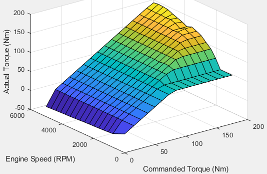Click to plot table.

#### Dependencies

To enable this parameter, clear Input engine temperature.

The engine torque lookup table is a function of commanded engine torque, engine speed, and engine temperature, T = ƒ(Tcmd, N, TempEng), where:

• T is engine torque, in N·m.

• Tcmd is commanded engine torque, in N·m.

• N is engine speed, in rpm.

• TempEng is engine temperature, in K.

#### Dependencies

To enable this parameter, select Input engine temperature.

Air

The engine air mass flow lookup table is a function of commanded engine torque and engine speed, ${\stackrel{˙}{m}}_{intk}$ = ƒ(Tcmd, N), where:

• ${\stackrel{˙}{m}}_{intk}$ is engine air mass flow, in kg/s.

• Tcmd is commanded engine torque, in N·m.

• N is engine speed, in rpm.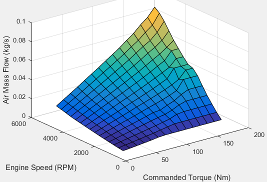#### Dependencies

To enable this parameter, clear Input engine temperature.

Click to plot table.

#### Dependencies

To enable this parameter, clear Input engine temperature.

The engine air mass flow lookup table is a function of commanded engine torque, engine speed, and engine temperature, ${\stackrel{˙}{m}}_{intk}$ = ƒ(Tcmd, N, TempEng), where:

• ${\stackrel{˙}{m}}_{intk}$ is engine air mass flow, in kg/s.

• Tcmd is commanded engine torque, in N·m.

• N is engine speed, in rpm.

• TempEng is engine temperature, in K.

#### Dependencies

To enable this parameter, select Input engine temperature.

Fuel

The engine fuel mass flow lookup table is a function of commanded engine torque and engine speed, MassFlow = ƒ(Tcmd, N), where:

• MassFlow is engine fuel mass flow, in kg/s.

• Tcmd is commanded engine torque, in N·m.

• N is engine speed, in rpm.#### Dependencies

To enable this parameter, clear Input engine temperature.

Click to plot table.

#### Dependencies

To enable this parameter, clear Input engine temperature.

The engine fuel mass flow lookup table is a function of commanded engine torque, engine speed, and engine temperature, MassFlow = ƒ(Tcmd, N, TempEng), where:

• MassFlow is engine fuel mass flow, in kg/s.

• Tcmd is commanded engine torque, in N·m.

• N is engine speed, in rpm.

• TempEng is engine temperature, in K.

#### Dependencies

To enable this parameter, select Input engine temperature.

Temperature

The engine exhaust temperature lookup table is a function of commanded engine torque and engine speed, Texh = ƒ(Tcmd, N), where:

• Texh is exhaust temperature, in K.

• Tcmd is commanded engine torque, in N·m.

• N is engine speed, in rpm.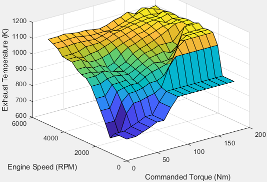#### Dependencies

To enable this parameter, clear Input engine temperature.

Click to plot table.

#### Dependencies

To enable this parameter, clear Input engine temperature.

The engine exhaust temperature lookup table is a function of commanded engine torque, engine speed, and engine temperature, Texh = ƒ(Tcmd, N, TempEng), where:

• Texh is exhaust temperature, in K.

• Tcmd is commanded engine torque, in N·m.

• N is engine speed, in rpm.

• TempEng is engine temperature, in K.

#### Dependencies

To enable this parameter, select Input engine temperature.

Efficiency

The brake-specific fuel consumption (BSFC) efficiency is a function of commanded engine torque and engine speed, BSFC = ƒ(Tcmd, N), where:

• BSFC is BSFC, in g/kWh.

• Tcmd is commanded engine torque, in N·m.

• N is engine speed, in rpm.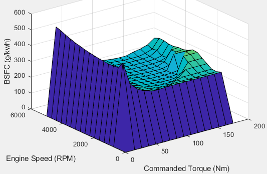#### Dependencies

To enable this parameter, clear Input engine temperature.

Click to plot table.

#### Dependencies

To enable this parameter, clear Input engine temperature.

The brake-specific fuel consumption (BSFC) efficiency is a function of commanded engine torque, engine speed, and engine temperature, BSFC = ƒ(Tcmd, N, TempEng), where:

• BSFC is BSFC, in g/kWh.

• Tcmd is commanded engine torque, in N·m.

• N is engine speed, in rpm.

• TempEng is engine temperature, in K.

#### Dependencies

To enable this parameter, select Input engine temperature.

HC

The engine-out hydrocarbon emissions are a function of commanded engine torque and engine speed, EO HC = ƒ(Tcmd, N), where:

• EO HC is engine-out hydrocarbon emissions, in kg/s.

• Tcmd is commanded engine torque, in N·m.

• N is engine speed, in rpm.#### Dependencies

To enable this parameter, clear Input engine temperature.

Click to plot table.

#### Dependencies

To enable this parameter, clear Input engine temperature.

The engine-out hydrocarbon emissions are a function of commanded engine torque, engine speed, and engine temperature, EO HC = ƒ(Tcmd, N, TempEng), where:

• EO HC is engine-out hydrocarbon emissions, in kg/s.

• Tcmd is commanded engine torque, in N·m.

• N is engine speed, in rpm.

• TempEng is engine temperature, in K.

#### Dependencies

To enable this parameter, select Input engine temperature.

CO

The engine-out carbon monoxide emissions are a function of commanded engine torque and engine speed, EO CO = ƒ(Tcmd, N), where:

• EO CO is engine-out carbon monoxide emissions, in kg/s.

• Tcmd is commanded engine torque, in N·m.

• N is engine speed, in rpm.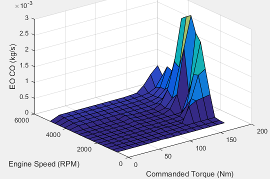#### Dependencies

To enable this parameter, clear Input engine temperature.

Click to plot table.

#### Dependencies

To enable this parameter, clear Input engine temperature.

The engine-out hydrocarbon emissions are a function of commanded engine torque, engine speed, and engine temperature, EO HC = ƒ(Tcmd, N, TempEng), where:

• EO HC is engine-out hydrocarbon emissions, in kg/s.

• Tcmd is commanded engine torque, in N·m.

• N is engine speed, in rpm.

• TempEng is engine temperature, in K.

#### Dependencies

To enable this parameter, select Input engine temperature.

NOx

The engine-out nitric oxide and nitrogen dioxide emissions are a function of commanded engine torque and engine speed, EO NOx = ƒ(Tcmd, N), where:

• EO NOx is engine-out nitric oxide and nitrogen dioxide emissions, in kg/s.

• Tcmd is commanded engine torque, in N·m.

• N is engine speed, in rpm.#### Dependencies

To enable this parameter, clear Input engine temperature.

Click to plot table.

#### Dependencies

To enable this parameter, clear Input engine temperature.

The engine-out nitric oxide and nitrogen dioxide emissions are a function of commanded engine torque, engine speed, and engine temperature, EO NOx = ƒ(Tcmd, N, TempEng), where:

• EO NOx is engine-out nitric oxide and nitrogen dioxide emissions, in kg/s.

• Tcmd is commanded engine torque, in N·m.

• N is engine speed, in rpm.

• TempEng is engine temperature, in K.

#### Dependencies

To enable this parameter, select Input engine temperature.

CO2

The engine-out carbon dioxide emissions are a function of commanded engine torque and engine speed, EO CO2 = ƒ(Tcmd, N), where:

• EO CO2 is engine-out carbon dioxide emissions, in kg/s.

• Tcmd is commanded engine torque, in N·m.

• N is engine speed, in rpm.#### Dependencies

To enable this parameter, clear Input engine temperature.

Click to plot table.

#### Dependencies

To enable this parameter, clear Input engine temperature.

The engine-out carbon dioxide emissions are a function of commanded engine torque, engine speed, and engine temperature, EO CO2 = ƒ(Tcmd, N, TempEng), where:

• EO CO2 is engine-out carbon dioxide emissions, in kg/s.

• Tcmd is commanded engine torque, in N·m.

• N is engine speed, in rpm.

• TempEng is engine temperature, in K.

#### Dependencies

To enable this parameter, select Input engine temperature.

PM

The engine-out particulate matter emissions are a function of commanded engine torque and engine speed, where:

• EO PM is engine-out PM emissions, in kg/s.

• Tcmd is commanded engine torque, in N·m.

• N is engine speed, in rpm.

#### Dependencies

To enable this parameter, clear Input engine temperature.

Click to plot table.

#### Dependencies

To enable this parameter, clear Input engine temperature.

The engine-out particulate matter emissions are a function of commanded engine torque, engine speed, and engine temperature, where:

• EO PM is engine-out PM emissions, in kg/s.

• Tcmd is commanded engine torque, in N·m.

• N is engine speed, in rpm.

• TempEng is engine temperature, in K.

#### Dependencies

To enable this parameter, select Input engine temperature.

## Version History

Introduced in R2017a